## Invention of Electronic Digital Computing

John L. Gustafson

ClearSpeed Technology, Inc.

### Abstract

The task that motivated Atanasoff’s construction of the first electronic digital computer has a mathematical legacy going back thousands of years, and it remains the fundamental operation by which computers are measured; solving systems of linear equations. This is sometimes misconstrued as a “special purpose” form of computing, but is actually as general in application as is any basic block of source code involving the four arithmetic operations +, –, ´, and ¸. Systems built for this function share many architectural features; 70 years after Atanasoff conceived the ABC, ClearSpeed is building hardware for the solution of the exact same type of calculation, but trillions of times faster. The quest for linear solvers has motivated some of the most important innovations in computing history.

#### 1. Introduction: A 2300-year-old quest

The solution of linear equations has been a primary goal for computation since the time of the abacus. Some examples of historical efforts follow.

1.1. 300 BC: Babylonian “word problems”

The following example, from a Babylonian clay tablet circa 300 BC, could have come from a modern-day text on beginning algebra:

There are two fields whose total area is 1800 square yards. One produces grain at the rate of 2/3 of a bushel per square yard while the other produces grain at the rate of 1/2 a bushel per square yard. If the total yield is 1100 bushels, what is the size of each field?

That tablet  may be the first record we have of the human need for linear equation solvers.

Those word problems also date from almost exactly the time of the earliest surviving calculating device , a “counting board” that worked like an abacus but with gravity holding pebbles in grooves instead of beads held on wires. The “Salamis Tablet,” circa 300 BC, may well have been used to solve those early sets of linear equations.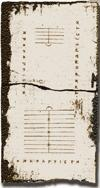Figure 1. The Salamis Tablet

By moving pebbles between columns of grooves on the white marble surface, this device aided accurate addition and subtraction up to 10,000; in modern terms, it was a four-decimal calculator. Note the dual registers; the top one has lower precision, such as might be required for the result of division operations on integers through repeated subtraction.

1.2. The Jiuzhang Suanshu: “calculation by square tables”

By 200 BC, Chinese mathematics rivaled and often surpassed that of Babylonia. The Jiuzhang Suanshu, or Nine Chapters on the Mathematical Art, dates from the Han Dynasty , yet includes as Chapter 8 a perfect description of what we now know as Gaussian elimination, with 18 examples including one with six equations in six unknowns! Perhaps “square table” is better translated as “matrix.” The examples show reduction to triangular form by scaling and subtraction, including the concept of negative numbers as partial results. The examples used small integers, but a 6 by 6 problem requires over 200 arithmetic operations that probably still took several hours to perform accurately with the help of an abacus.

1.3. Two millennia pass

From the Chinese achievement to the time of Gauss, we see only records of 2 by 2 and 3 by 3 systems of equations, where the solution methods are various ways of expressing Cramer’s Rule. Cramer’s Rule is elegant for such small systems, immediately informing the user if the system is insolvable (by concluding in a division by zero), but scales as the factorial of the number of equations. Even to solve a 4 by 4 system exceeds the patience of most people, particularly since Cramer’s Rule tends to create many-decimal intermediate results from simple starting coefficients. It appears that the fundamental technique described in the Jiuzhang Suanshu was lost for almost 2000 years.

#### 1.4. Gauss and the asteroids Ceres and Pallas

In 1801 and 1802, astronomers spotted the first asteroids (named “minor planets” at the time). Named Ceres and Pallas, predicting their orbits obsessed the great mathematician Carl Friedrich Gauss. In both cases, the asteroids provided only a few position measurements before disappearing in the glare of the sun.Figure 2. The asteroid Pallas

Gauss’ mathematical colleagues watched in horror  as the genius, having made so many breakthroughs in fundamental mathematics, set abstract theory aside and threw himself into several years of tedious arithmetic calculations to test a physical theory about where they would next be observed. But Gauss, as usual, was right in his choice of what was and wasn’t important to study. To solve the problem of predicting the orbit of Pallas, he had to reduce astronomical data to a least-squares problem… involving six equations in six unknowns. It was the first application of the method of least squares, a cornerstone of statistical analysis he had invented a few years earlier. It led him to describe the system for solving linear systems in general that bears his name, simultaneously advancing statistics, celestial mechanics, and numerical methods in far-reaching ways.

1.5. Babbage’s Analytical Engine (1836)

Many of us can recall grand plans for supercomputers that did not become reality. Often, however, the mere act of working out the details on paper allows major advances in technology. Charles Babbage’s ambitious plan for a mechanical (steam-powered) digital computer was precise and detailed enough that Ada Lovelace was able to write programs for the nonexistent system.

One of her first programs was for solution of a system of ten linear equations in ten unknowns . She used a concept of resetting the card containing the instruction to perform a repeated operation on a list of numbers economically; it was the first vector instruction, invented specifically for the centuries old desire to solve Ax = b. She was not only the first computer programmer by 110 years; she was about 150 years ahead of her time in wanting to use a supercomputer to run LINPACK! Incidentally, the Analytical engine took two to four minutes to do a multiply-add operation, so Ada’s program would have taken about 60 hours to solve the 10 equations. She once said the looping effect could “solve a system of linear equations no matter how big it was” .

The committee that reviewed Babbage’s plans for the machine had this to say :

Another important desideratum to which the machine might be adapted… is the solution of simultaneous equations containing many variables. This would include a large part of the calculations involved in the method of least squares… In the absence of a special engine for the purpose, the solution of large sets of simultaneous equations is a most laborious task, and a very expensive process indeed, when it has to be paid for, in the cases in which the result is imperatively needed.

But the machine was not to be. It would be another century before the first working automatic linear equation solver.

1.6 The Atanasoff-Berry Computer (ABC)

Atanasoff may have been the first to estimate a general “LINPACK” rate for a human calculator in his 1940 paper :

It is easy to see that the principal term in the amount of labor needed to solve a system of equations is kN3 in which N is the number of unknowns and k is a constant. Since an expert [human] computer requires about eight hours to solve a full set of eight equations in eight unknowns, k is about 1/64.

This implies a calculation rate of 0.016 flops/s, or almost exactly one minute per multiply or add. (We use “flops” for the plural of “floating-point operation” and “flops/s” as the abbreviation for the speed measure, to avoid confusion.) He was probably assuming the use of 10-decimal mechanical calculators such as those made by Marchant or Monroe to assist the hand calculation; unaided pencil-and-paper arithmetic is about ten times slower than this.

By using 30 processors in parallel, the ABC could claim a peak speed of 30 adds per second with 15-decimal precision. However, our timings of the use of the machine to solve linear equations including all human setup and I/O showed the sustained speed to be about 0.06 operations per second. This was still four times faster than doing the calculation manually, higher precision, and far less error-prone since the ABC recorded intermediate results automatically.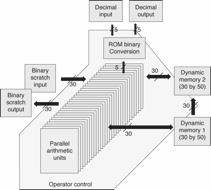Figure 3. ABC diagram

The reason Atanasoff felt linear equations were so important bears repeating. Here is his list of examples to which he wanted to apply the kernel operation:

1. Multiple correlation

2. Curve fitting

3. Method of least squares

4. Vibration problems including the vibrational
Raman effect

5. Electrical circuit analysis

6. Analysis of elastic structures

7. Approximate solution of many problems of elasticity

8. Approximate solution of problems of quantum mechanics

9. Perturbation theories of mechanics, astronomy, and quantum theory

The first three are paraphrases of statistical fitting problems for which Iowa State College had a strong applied statistics presence, and remind us of the reason Gauss invented those methods. The other items on this list are even more prescient, reminding us of modern HPC programs like SPICE, ANSYS, and GAUSSIAN.

2. Is equation solving “special purpose”?

Few terms in computer technology are used as carelessly as “special purpose,” (though “real time” is also a contender.) As commonly used, a “special purpose” computer is better than we expect at some things, and not as good as we expect at other things, where the expectations are set simply by prior experience. The laptop on which I am typing this is very poorly suited to driving a car automatically, for example, yet few would call it “special purpose.”

2.1 Was the ABC a general-purpose computer?

Those who seek to minimize the importance of the ABC often label it “special purpose.” This attitude shows a misunderstanding of how general the power of linear solver hardware is.

In solving a simple system of two equations in two unknowns on the ABC replica, we noticed that the machine produced the result dbc⁄a in the lower right storage location, just as someone solving the system by hand would produce it. However, that meant that the ABC was a complete four-function calculator! Specifically:

Table 1. 2x2 entries that yield basic math

 To compute a b c d X + Y 1 Y –1 X X – Y 1 Y 1 X X ´ Y 1 X Y 0 X ¸ Y Y X –1 0

There is a bit of this idea in some instruction sets that use the “fused multiply-add” instruction. This computes p = ´ s in hardware, but if all you want is an addition or a multiplication, you simply use it with = 0 or = 1 drawn from dedicated registers containing those values.

We can easily extend this idea by considering the solution of n equations in n unknowns as a template into which we may embed any sequence of arithmetic operations. For example, suppose we wish to compute the first few terms of the Taylor series for ex: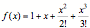The ABC (or any other Gaussian elimination hardware) computes this f(x) if we simply set up a 3 by 3 matrix as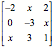,

and solve for upper triangular form, The f(x) value appears in the lower right-hand corner.

2.2. Was ENIAC a “special purpose” computer?

Embedding arithmetic sequences into a linear solve may seem like a strange way to “program” a function, but the system for other computers that followed the ABC were scarcely less strange. The ENIAC was “programmed” by removing and re-attaching wires between functional units, and then manually setting three thousand switches on the function tables . Whereas the computation of ballistic tables took a single person twenty hours to do with a hand calculator, the ENIAC required a team of six operator-programmers and about seventy hours, if you include the time to set up the computer for that problem.

The ENIAC did time-stepped systems of ordinary differential equations with three unknowns. It would not have been difficult to perform the same calculations with the ABC by the method described in §2,1. Oddly enough, even with 18,000 vacuum tubes, the ENIAC could only hold 20 10-digit numbers, fewer than the ABC’s 60 15-digit numbers. We have no record of the ENIAC ever having been programmed to solve a system of linear equations, despite von Neumann’s intense interest in using linear solvers for fluid dynamics problems. Since the process of changing the ENIAC circuitry and switches was extremely expensive and error-prone, the machine was usually left in a single dataflow pattern for many months. Once again, we see that the terms “special purpose” and “general purpose” are often applied thoughtlessly. By any definition that takes into account the human effort needed to change the purpose of a machine, the ENIAC was very special-purpose, certainly more so than the ABC.

2.3 Linear solvers and the ACRITH package

The generality of linear equation solvers is the basis for IBM’s ACRITH and Pascal-XSC for very high-precision arithmetic. The concept, due to Kulisch , as to convert a basic block of operations to a linear system of equations, which is solved using an extended-precision accumulator.  Since square roots, trigonometric functions, etc, all break down to the four fundamental arithmetic operations within library routines (or low-level hardware), the only remaining computing element is the management of control flow (branching, subroutine calls) and input/output.

This modern tool for precise computation, commercialized as PASCAL-XSC and ACRITH programming environments, illustrates both the power and generality of linear equations for solving a wide range of problems.

3. The rise of LINPACK and the TOP500

Fast computers immediately raised serious concerns about solving large linear system with floating-point arithmetic that rounded results. Both Hotelling and Turing independently concluded that the rounding errors would grow exponentially with the number of equations . By 1961, Wilkinson put the concerns were put to rest; he proved the adequacy of Gaussian elimination if one is careful to choose the sequence of rows used to eliminate the other rows. This choice, called “pivoting,” removed one of the two obvious hurdles in the quest for large linear solvers. (Wilkinson had participated in the creation of the ACE computer, intended for the solution of 18 equations in 18 unknowns.)

The other hurdle, of course, was speed. Then as now, users had no trouble conceiving of problems far larger than they could solve in practical amounts of time. Since the computers of the 1950s took far longer to do a high precision multiply and add than just about any other operation, the numerical analysis community measured the difficulty of a task by how many “flops” it required.

Building on Wilkinson’s work, Dongarra et al.  created a project to create high-quality software for linear algebra, and then disseminate it free of charge. It probably was the first “open source” project, and it revolutionized the way programmers developed and distributed essential algorithms to the computing community. As an unassuming note near the end of the 1970s “LINPACK Users Guide” the authors give examples of the speeds of a few commercially-available systems using the software, and an invitation to send other speed measurements to Dongarra. Thus began the best-known and longest-lasting benchmark in computer history.

The original LINPACK benchmark problem was for 100 unknowns. As computers got faster, Dongarra introduced a 300 by 300 problem, which by requiring almost 30 times as much arithmetic to solve, took care of Moore’s law improvements for a few years. Even this began to look too small, so Dongarra added a 1000-equation case as a separate benchmark. Around 1984, I suggested to Dongarra that he permit the problem to scale, allowing any linear system size and then ranking simply on the achieved flops/s. And, by defining the problem as “Solve a system of equations with Gaussian elimination using partial pivoting,” the problem need not be tied to any particular source code or presumed architecture. Dongarra adopted these ideas in a separate list, “Toward Peak Performance,” and soon had hundreds of entries. Vendors struggled to achieve a high ranking on the list, to the point of making architectural choices based on how it might improve their rank.

Though created as a mathematical library, the use of LINPACK as a benchmark had become an institution. It was codified at the TOP500 list (www.top500.org) in the early 1990s and remains the way we track the progress of computer technology.

4. Modern Architectures for Linear Solvers

A “sea change” occurred in computer architectures not long after the LINPACK library was introduced: flops were becoming cheaper and faster than memory references. This is counterintuitive to us, and still surprising to many, because a human can write down a 15-decimal arithmetic problem in just a few seconds that will take at least ten minutes to solve with pencil and paper methods. Around 1975–1977, computers started taking almost exactly the same time to perform a 64-bit multiply-add as they did to fetch and store the operands. Yet, the tendency to measure computational “work” by counting the flops persists to this day in many circles.

Once VLSI technology advanced to the point of putting a full 64-bit floating-point multiplier and adder on one chip, it was only a matter of time before a company built a platform that exploited the fact that linear equation solving does far more arithmetic than fetching and storing.

4.1 The FPS-164/MAX

By 1984, dense linear solvers had shown their importance particularly in three major areas: radar simulation, structural analysis, and computational chemistry. Other fields, such as analog circuit simulation (SPICE), used linear systems that were so sparse that they had  closer to one-to-one ratio of arithmetic to communication, but the dense problems had an attractive feature: order N3 arithmetic but only order N2 data motion. At FPS, we set out to build a system that was stunningly faster (341 Mflops/s) than anything but the largest Cray mainframe, by exploiting the new rules of architectural balance that VLSI floating-point math had made possible .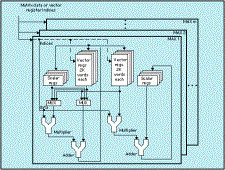Figure 4. The FPS-164/MAX accelerators

We were not aware of the ABC or its architecture. Only after the product was designed and shipping did a colleague, Edward Borasky, point out a paper by one J. V. Atanasoff, dated 1940. We, too, had elected to use 30 multiply-add units in parallel under a single stream of instruction control, 15-decimal precision, and many other features that Atanasoff had chosen 44 years earlier. We were astonished by how far ahead of his time his design was.

4.2 The ClearSpeed CSX600

In early 2005, ClearSpeed Technology presented me a striking piece of technology: A chip capable of sustaining about 25 Gflops/s, while consuming less than 10 watts. And it did best on algorithms that perform a very high ratio of arithmetic to memory transfers. It was particularly good at matrix-matrix multiplication, for example, just like the FPS-164/MAX had been, and like the FPS system, that capability was applied immediately to the solution of large systems of linear equations.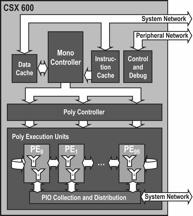Figure 5. The ClearSpeed accelerator

The ClearSpeed designers had never heard of the ABC, nor did they know about the FPS-164/MAX, yet they had followed in the footsteps of both computers. The ClearSpeed CSX600 chip had 96 multiplier-adder units under a single stream of instruction control.

I have been very fortunate to work on the ABC, the FPS-164/MAX, and the ClearSpeed CSX600… three architectures that have all recognized the importance of linear solvers, spanning over six decades of technology advances. The ClearSpeed board is approximately a trillion times faster than the ABC, yet is often used to perform the exact same task with the same number of decimals of precision, allowing us that direct speed comparison of the ABC with the ClearSpeed chip. While Moore’s law was a statement about density and price improvements in large-scale integration, it is often cited as an estimate for speed improvement as well. We can even compute the “Moore’s law” for the 65-year time of introduction of the two devices (1940 and 2005) at 53% per year. The usual guideline of “doubles every 18 months” works out to 59% per year.

5 The New Challenges for Linear Solvers

At the time of this writing, about a dozen institutions are striving to build supercomputer clusters capable of delivering over 1.0 Pflops/s at LINPACK. The obstacles that now come into the foreground are power consumption (and heat removal), reliability of such massive amounts of hardware, and coordination of operating systems to avoid “OS jitter” that interferes with smooth parallel computation. The ClearSpeed product, in particular, was designed to solve the power consumption problem for supercomputing. It appears that the next generation of top-end systems will use “hybrid” computing methods like ClearSpeed that use coprocessors so that each type of computing task runs on the hardware best suited for that function.

The efforts to solve these problems for large LINPACK runs cause technical advances that then benefit every application for which the system is used.

6 Conclusions

For over 2000 years, there has been a remarkable common thread through advances in computing technology: The quest for linear equation solvers. We see

• The invention of matrices and Gaussian elimination

• Vector arithmetic hardware

• The first electronic digital computer

• Seminal and enabling works in numerical analysis

• The first open-source computing project

• An international standard for ranking computers

as having been motivated by this ancient challenge.

The legacy continues to this day as vendors and customers struggle for ever faster results on the TOP500, not so much because they need to solve gigantic systems of linear equations as part of their workload but because the task it represents is so fundamental to what people have always sought from automatic computing devices.

If you can solve a system of linear equations on a modern supercomputer, it means you have solved a lot more than the equations. It means you have solved the issues of rounding error, interprocessor communication bandwidth and latency, reliability, algorithm parallelization, precision sufficiency, process synchronization, OS jitter, management of a many-tiered memory hierarchy, and lately even issues of power consumption and heat dissipation. The Atanasoff-Berry Computer certainly was not “special purpose,” it solved the problem that remains the primary task of scientific computing. n

References

    Jennings, A., “Matrices, Ancient and Modern,” Bull. Inst. Math. Appl., 13 (5) (1977), pp.117–123.

    Fernandes, Luis (2003) A Brief History of The Abacus

    Mo, S.K., “Jiuzhang Suanshu” (“Nine chapters on the mathematical art”) and Liu Hui's commentary (Chinese), Stud. Hist. Nat. Sci. 19 (2) (2000), pp. 97–113.

    Bell, E. T., reprinted in The World of Mathematics, Vol. 1., J. R. Newman, ed., Dover Publications, 2003.

    Cayley et al., “Report of the Committee… to consider the advisability and to estimate the expense of constructing Mr. Babbage’s Analytical Machine, and of printing Tables by its means,” reprinted in The Origins of Digital Computers, B. Randell, ed., Third Edition, Springer-Verlag, New York, 1982, p. 64.

    Falbo, C., <http://www.sonoma.edu/Math/faculty/falbo/

blaugustalovelace.htm
>, Dec. 2000.

    Atanasoff, J. V., “Computing Machine for the Solution of Large Systems of Linear Algebraic Equations,” reprinted in The Origins of Digital Computers, B. Randell, ed., 3rd Edition, Springer-Verlag, New York, 1982, pp. 315–335.

    Kopplin, J., “Illustrated History of Computers Part 4” <http://www.computersciencelab.com/ComputerHistory/HistoryPt4.htm>

 Kulisch, U., and Miranker, W. L., A New Approach to Scientific Computation, Academic Press, 1983.

 Parlett, B., “Very Early Days of Matrix Computations,” SIAM News, Vol. 36, No. 9, Nov. 2003.

 Dongarra, J. J., Bunch, C., Moler, C., and Stewart, G., LINPACK Users Guide, SIAM Press, Philadelphia, 1979.

 Charlesworth, A., and Gustafson, J., “Introducing Replicated VLSI to Supercomputing: The FPS-164/MAX Scientific Computer,” IEEE Computer, March 1986.